# 看我如何编写一个验证码识别程序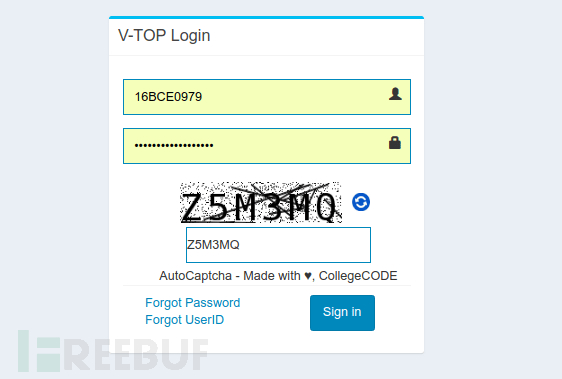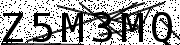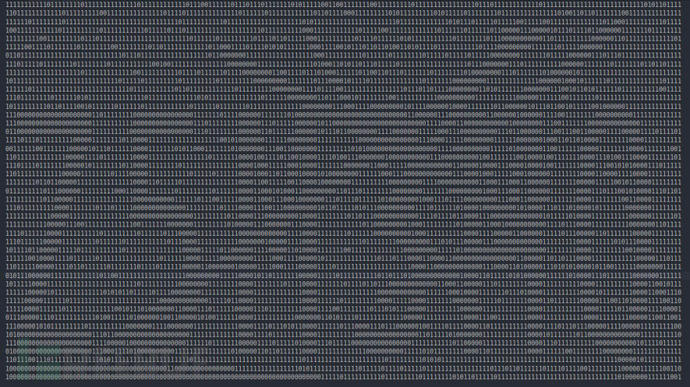`from PIL import Image image = Image.open("filename.xyz") cropped_image = image.crop((left, upper, right, lower))`

`from PIL import Image image = Image.open("captcha.png").convert("L") # Grayscale conversion cropped_image = image.crop((0, 0, 30, 45)) cropped_image.save("cropped_image.png")`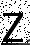`pixel_matrix = cropped_image.load() for col in range(0, cropped_image.height): for row in range(0, cropped_image.width): if pixel_matrix[row, col] != 0: pixel_matrix[row, col] = 255image.save("thresholded_image.png")``for column in range(1, image.height - 1): for row in range(1, image.width - 1): if pixel_matrix[row, column] == 0 \ and pixel_matrix[row, column - 1] == 255 and pixel_matrix[row, column + 1] == 255 : pixel_matrix[row, column] = 255 if pixel_matrix[row, column] == 0 \ and pixel_matrix[row - 1, column] == 255 andpixel_matrix[row + 1, column] == 255: pixel_matrix[row, column] = 255`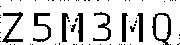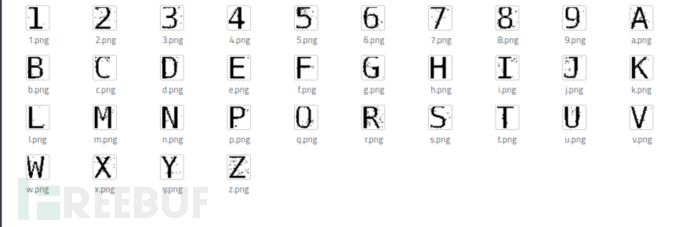`import json characters = "123456789abcdefghijklmnpqrstuvwxyz" captcha = "" with open("bitmaps.json", "r") asf: bitmap = json.load(f) for j in range(image.width/6, image.width + 1, image.width/6): character_image = image.crop((j - 30, 12, j, 44)) character_matrix = character_image.load() matches = {} for char in characters: match = 0 black = 0 bitmap_matrix = bitmap[char] for y in range(0, 32): for x in range(0, 30): ifcharacter_matrix[x, y] == bitmap_matrix[y][x] and bitmap_matrix[y][x] == 0: match += 1 if bitmap_matrix[y][x] == 0: black += 1 perc = float(match) / float(black) matches.update({perc: char.upper()}) try: captcha += matches[max(matches.keys())] except ValueError: print("failed captcha") captcha += "0" print captcha`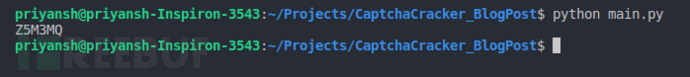Tags：验证码识别程序编程入门

• 文章总数:102
• 页面总数:3
• 分类总数:29
• 标签总数:275
• 评论总数:6
• 浏览总数:10037